# Variations on the Higman's Lemma

Aug. 8, 2016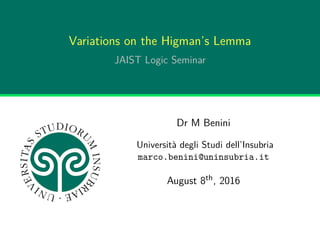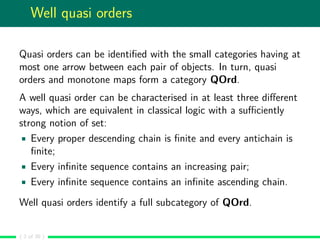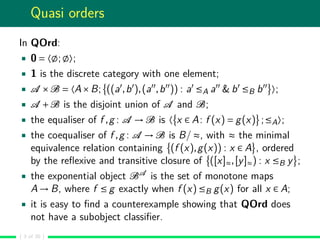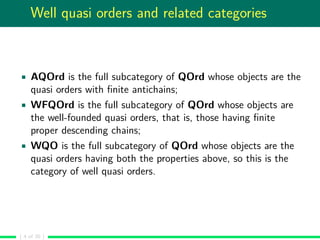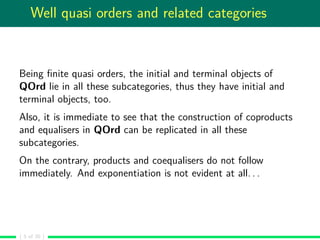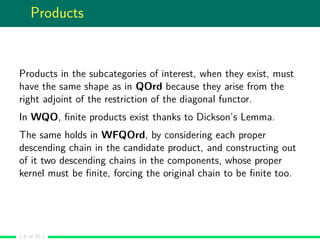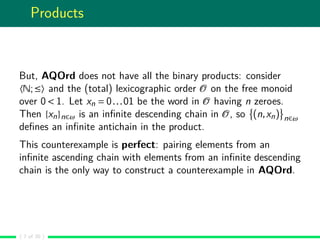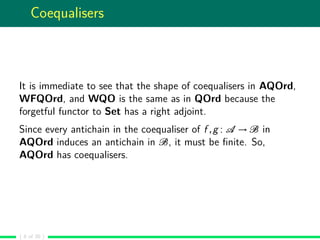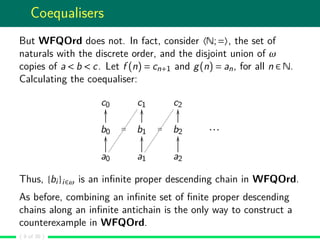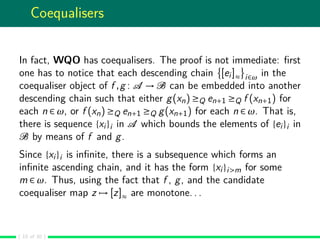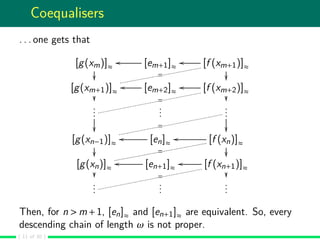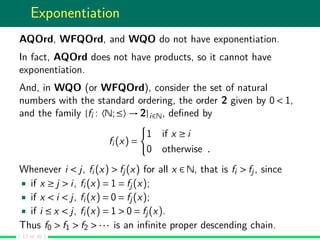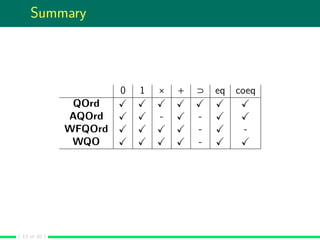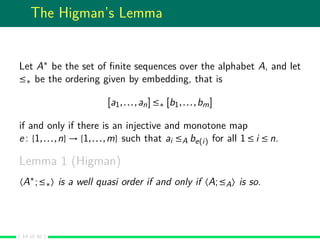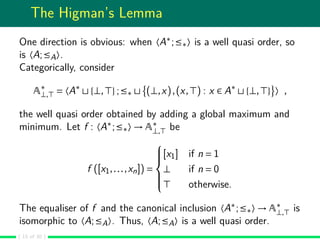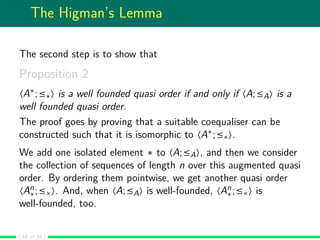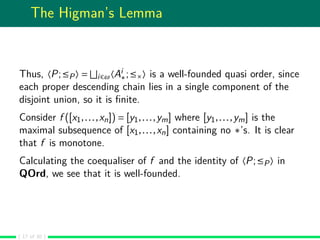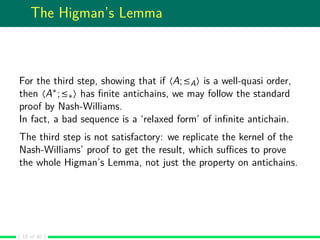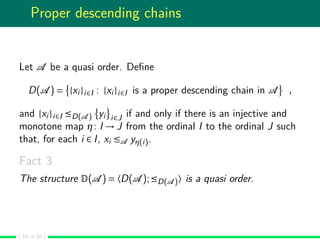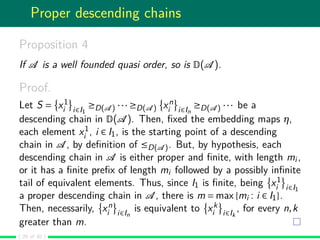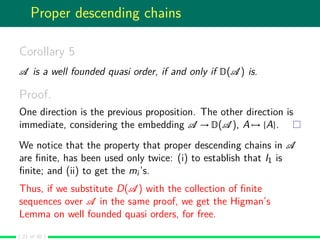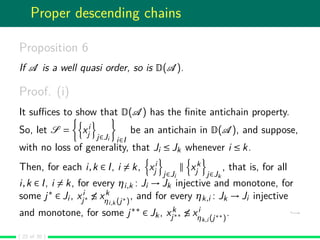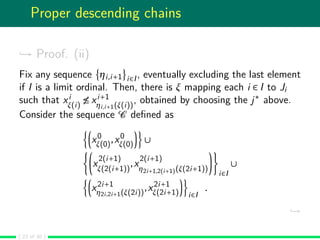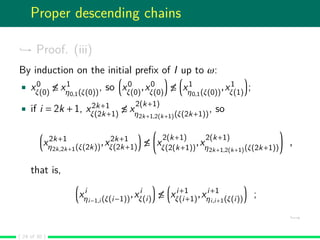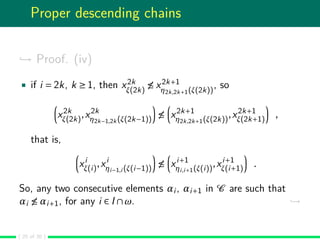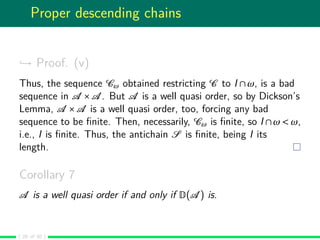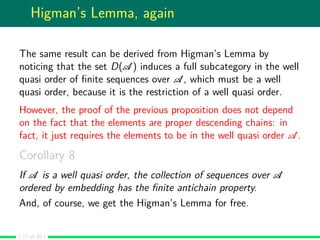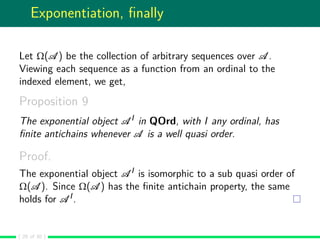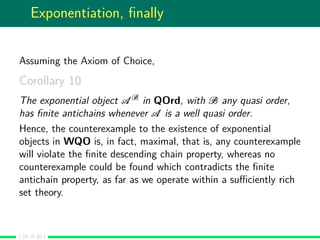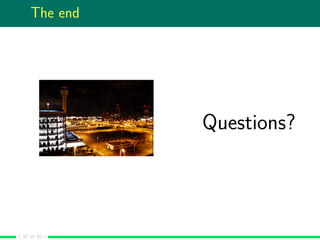1 of 30

### Variations on the Higman's Lemma

1. Variations on the Higman’s Lemma JAIST Logic Seminar Dr M Benini Università degli Studi dell’Insubria marco.benini@uninsubria.it August 8th, 2016
2. Well quasi orders Quasi orders can be identiﬁed with the small categories having at most one arrow between each pair of objects. In turn, quasi orders and monotone maps form a category QOrd. A well quasi order can be characterised in at least three diﬀerent ways, which are equivalent in classical logic with a suﬃciently strong notion of set: Every proper descending chain is ﬁnite and every antichain is ﬁnite; Every inﬁnite sequence contains an increasing pair; Every inﬁnite sequence contains an inﬁnite ascending chain. Well quasi orders identify a full subcategory of QOrd. ( 2 of 30 )
3. Quasi orders In QOrd: 0 = 〈 ; 〉; 1 is the discrete category with one element; A ×B = 〈A×B; ((a ,b ),(a ,b )) : a ≤A a & b ≤B b 〉; A +B is the disjoint union of A and B; the equaliser of f ,g : A → B is 〈 x ∈ A: f (x) = g(x) ;≤A〉; the coequaliser of f ,g : A → B is B/ ≈, with ≈ the minimal equivalence relation containing (f (x),g(x)) : x ∈ A , ordered by the reﬂexive and transitive closure of ([x]≈,[y]≈) : x ≤B y ; the exponential object BA is the set of monotone maps A → B, where f ≤ g exactly when f (x) ≤B g(x) for all x ∈ A; it is easy to ﬁnd a counterexample showing that QOrd does not have a subobject classiﬁer. ( 3 of 30 )
4. Well quasi orders and related categories AQOrd is the full subcategory of QOrd whose objects are the quasi orders with ﬁnite antichains; WFQOrd is the full subcategory of QOrd whose objects are the well-founded quasi orders, that is, those having ﬁnite proper descending chains; WQO is the full subcategory of QOrd whose objects are the quasi orders having both the properties above, so this is the category of well quasi orders. ( 4 of 30 )
5. Well quasi orders and related categories Being ﬁnite quasi orders, the initial and terminal objects of QOrd lie in all these subcategories, thus they have initial and terminal objects, too. Also, it is immediate to see that the construction of coproducts and equalisers in QOrd can be replicated in all these subcategories. On the contrary, products and coequalisers do not follow immediately. And exponentiation is not evident at all. . . ( 5 of 30 )
6. Products Products in the subcategories of interest, when they exist, must have the same shape as in QOrd because they arise from the right adjoint of the restriction of the diagonal functor. In WQO, ﬁnite products exist thanks to Dickson’s Lemma. The same holds in WFQOrd, by considering each proper descending chain in the candidate product, and constructing out of it two descending chains in the components, whose proper kernel must be ﬁnite, forcing the original chain to be ﬁnite too. ( 6 of 30 )
7. Products But, AQOrd does not have all the binary products: consider 〈N;≤〉 and the (total) lexicographic order O on the free monoid over 0 < 1. Let xn = 0...01 be the word in O having n zeroes. Then {xn}n∈ω is an inﬁnite descending chain in O, so (n,xn) n∈ω deﬁnes an inﬁnite antichain in the product. This counterexample is perfect: pairing elements from an inﬁnite ascending chain with elements from an inﬁnite descending chain is the only way to construct a counterexample in AQOrd. ( 7 of 30 )
8. Coequalisers It is immediate to see that the shape of coequalisers in AQOrd, WFQOrd, and WQO is the same as in QOrd because the forgetful functor to Set has a right adjoint. Since every antichain in the coequaliser of f ,g : A → B in AQOrd induces an antichain in B, it must be ﬁnite. So, AQOrd has coequalisers. ( 8 of 30 )
9. Coequalisers But WFQOrd does not. In fact, consider 〈N;=〉, the set of naturals with the discrete order, and the disjoint union of ω copies of a < b < c. Let f (n) = cn+1 and g(n) = an, for all n ∈ N. Calculating the coequaliser: c0 c1 c2 b0 OO b1 OO b2 OO ··· a0 OO = a1 OO = a2 OO Thus, {bi}i∈ω is an inﬁnite proper descending chain in WFQOrd. As before, combining an inﬁnite set of ﬁnite proper descending chains along an inﬁnite antichain is the only way to construct a counterexample in WFQOrd. ( 9 of 30 )
10. Coequalisers In fact, WQO has coequalisers. The proof is not immediate: ﬁrst one has to notice that each descending chain [ei]≈ i∈ω in the coequaliser object of f ,g : A → B can be embedded into another descending chain such that either g(xn) ≥Q en+1 ≥Q f (xn+1) for each n ∈ ω, or f (xn) ≥Q en+1 ≥Q g(xn+1) for each n ∈ ω. That is, there is sequence {xi}i in A which bounds the elements of {ei}i in B by means of f and g. Since {xi}i is inﬁnite, there is a subsequence which forms an inﬁnite ascending chain, and it has the form {xi}i>m for some m ∈ ω. Thus, using the fact that f , g, and the candidate coequaliser map z → [z]≈ are monotone. . . ( 10 of 30 )
11. Coequalisers . . . one gets that [g(xm)]≈ [em+1]≈ oo [f (xm+1)]≈ oo [g(xm+1)]≈ = [em+2]≈ oo [f (xm+2)]≈ oo = ... ... ... [g(xn−1)]≈ = [en]≈ oo [f (xn)]≈ oo = [g(xn)]≈ = [en+1]≈ oo [f (xn+1)]≈ oo = ... ... ... Then, for n m+1, [en]≈ and [en+1]≈ are equivalent. So, every descending chain of length ω is not proper. ( 11 of 30 )
12. Exponentiation AQOrd, WFQOrd, and WQO do not have exponentiation. In fact, AQOrd does not have products, so it cannot have exponentiation. And, in WQO (or WFQOrd), consider the set of natural numbers with the standard ordering, the order 2 given by 0 1, and the family {fi : 〈N;≤〉 → 2}i∈N, deﬁned by fi(x) = 1 if x ≥ i 0 otherwise . Whenever i j, fi(x) fj(x) for all x ∈ N, that is fi fj, since if x ≥ j i, fi(x) = 1 = fj(x); if x i j, fi(x) = 0 = fj(x); if i ≤ x j, fi(x) = 1 0 = fj(x). Thus f0 f1 f2 ··· is an inﬁnite proper descending chain. ( 12 of 30 )
13. Summary 0 1 × + ⊃ eq coeq QOrd AQOrd - - WFQOrd - - WQO - ( 13 of 30 )
14. The Higman’s Lemma Let A∗ be the set of ﬁnite sequences over the alphabet A, and let ≤∗ be the ordering given by embedding, that is [a1,...,an] ≤∗ [b1,...,bm] if and only if there is an injective and monotone map e : {1,...,n} → {1,...,m} such that ai ≤A be(i) for all 1 ≤ i ≤ n. Lemma 1 (Higman) 〈A∗ ;≤∗〉 is a well quasi order if and only if 〈A;≤A〉 is so. ( 14 of 30 )
15. The Higman’s Lemma One direction is obvious: when 〈A∗ ;≤∗〉 is a well quasi order, so is 〈A;≤A〉. Categorically, consider A∗ ⊥, = 〈A∗ {⊥, };≤∗ (⊥,x),(x, ) : x ∈ A∗ {⊥, } 〉 , the well quasi order obtained by adding a global maximum and minimum. Let f : 〈A∗ ;≤∗〉 → A∗ ⊥, be f ([x1,...,xn]) =    [x1] if n = 1 ⊥ if n = 0 otherwise. The equaliser of f and the canonical inclusion 〈A∗ ;≤∗〉 → A∗ ⊥, is isomorphic to 〈A;≤A〉. Thus, 〈A;≤A〉 is a well quasi order. ( 15 of 30 )
16. The Higman’s Lemma The second step is to show that Proposition 2 〈A∗ ;≤∗〉 is a well founded quasi order if and only if 〈A;≤A〉 is a well founded quasi order. The proof goes by proving that a suitable coequaliser can be constructed such that it is isomorphic to 〈A∗ ;≤∗〉. We add one isolated element ∗ to 〈A;≤A〉, and then we consider the collection of sequences of length n over this augmented quasi order. By ordering them pointwise, we get another quasi order 〈An ∗;≤×〉. And, when 〈A;≤A〉 is well-founded, 〈An ∗;≤×〉 is well-founded, too. ( 16 of 30 )
17. The Higman’s Lemma Thus, 〈P;≤P〉 = i∈ω〈Ai ∗;≤×〉 is a well-founded quasi order, since each proper descending chain lies in a single component of the disjoint union, so it is ﬁnite. Consider f ([x1,...,xn]) = [y1,...,ym] where [y1,...,ym] is the maximal subsequence of [x1,...,xn] containing no ∗’s. It is clear that f is monotone. Calculating the coequaliser of f and the identity of 〈P;≤P〉 in QOrd, we see that it is well-founded. ( 17 of 30 )
18. The Higman’s Lemma For the third step, showing that if 〈A;≤A〉 is a well-quasi order, then 〈A∗ ;≤∗〉 has ﬁnite antichains, we may follow the standard proof by Nash-Williams. In fact, a bad sequence is a ‘relaxed form’ of inﬁnite antichain. The third step is not satisfactory: we replicate the kernel of the Nash-Williams’ proof to get the result, which suﬃces to prove the whole Higman’s Lemma, not just the property on antichains. ( 18 of 30 )
19. Proper descending chains Let A be a quasi order. Deﬁne D(A ) = {xi}i∈I : {xi}i∈I is a proper descending chain in A , and {xi}i∈I ≤D(A ) yi i∈J if and only if there is an injective and monotone map η: I → J from the ordinal I to the ordinal J such that, for each i ∈ I, xi ≤A yη(i). Fact 3 The structure D(A ) = 〈D(A );≤D(A )〉 is a quasi order. ( 19 of 30 )
20. Proper descending chains Proposition 4 If A is a well founded quasi order, so is D(A ). Proof. Let S = x1 i i∈I1 ≥D(A ) ··· ≥D(A ) xn i i∈In ≥D(A ) ··· be a descending chain in D(A ). Then, ﬁxed the embedding maps η, each element x1 i , i ∈ I1, is the starting point of a descending chain in A , by deﬁnition of ≤D(A ). But, by hypothesis, each descending chain in A is either proper and ﬁnite, with length mi, or it has a ﬁnite preﬁx of length mi followed by a possibly inﬁnite tail of equivalent elements. Thus, since I1 is ﬁnite, being x1 i i∈I1 a proper descending chain in A , there is m = max{mi : i ∈ I1}. Then, necessarily, xn i i∈In is equivalent to xk i i∈Ik , for every n,k greater than m. ( 20 of 30 )
21. Proper descending chains Corollary 5 A is a well founded quasi order, if and only if D(A ) is. Proof. One direction is the previous proposition. The other direction is immediate, considering the embedding A → D(A ), A → {A}. We notice that the property that proper descending chains in A are ﬁnite, has been used only twice: (i) to establish that I1 is ﬁnite; and (ii) to get the mi’s. Thus, if we substitute D(A ) with the collection of ﬁnite sequences over A in the same proof, we get the Higman’s Lemma on well founded quasi orders, for free. ( 21 of 30 )
22. Proper descending chains Proposition 6 If A is a well quasi order, so is D(A ). Proof. (i) It suﬃces to show that D(A ) has the ﬁnite antichain property. So, let S = xi j j∈Ji i∈I be an antichain in D(A ), and suppose, with no loss of generality, that Ji ≤ Jk whenever i ≤ k. Then, for each i,k ∈ I, i = k, xi j j∈Ji xk j j∈Jk , that is, for all i,k ∈ I, i = k, for every ηi,k : Ji → Jk injective and monotone, for some j∗ ∈ Ji, xi j∗ ≤ xk ηi,k (j∗) , and for every ηk,i : Jk → Ji injective and monotone, for some j∗∗ ∈ Jk, xk j∗∗ ≤ xi ηk,i (j∗∗) . → ( 22 of 30 )
23. Proper descending chains → Proof. (ii) Fix any sequence ηi,i+1 i∈I, eventually excluding the last element if I is a limit ordinal. Then, there is ξ mapping each i ∈ I to Ji such that xi ξ(i) ≤ xi+1 ηi,i+1(ξ(i)) , obtained by choosing the j∗ above. Consider the sequence C deﬁned as x0 ξ(0),x0 ξ(0) ∪ x 2(i+1) ξ(2(i+1)) ,x 2(i+1) η2i+1,2(i+1)(ξ(2i+1)) i∈I ∪ x2i+1 η2i,2i+1(ξ(2i)),x2i+1 ξ(2i+1) i∈I . → ( 23 of 30 )
24. Proper descending chains → Proof. (iii) By induction on the initial preﬁx of I up to ω: x0 ξ(0) ≤ x1 η0,1(ξ(0)) , so x0 ξ(0) ,x0 ξ(0) ≤ x1 η0,1(ξ(0)) ,x1 ξ(1) ; if i = 2k +1, x2k+1 ξ(2k+1) ≤ x 2(k+1) η2k+1,2(k+1)(ξ(2k+1)) , so x2k+1 η2k,2k+1(ξ(2k)),x2k+1 ξ(2k+1) ≤ x 2(k+1) ξ(2(k+1)) ,x 2(k+1) η2k+1,2(k+1)(ξ(2k+1)) , that is, xi ηi−1,i (ξ(i−1)),xi ξ(i) ≤ xi+1 ξ(i+1),xi+1 ηi,i+1(ξ(i)) ; → ( 24 of 30 )
25. Proper descending chains → Proof. (iv) if i = 2k, k ≥ 1, then x2k ξ(2k) ≤ x2k+1 η2k,2k+1(ξ(2k)) , so x2k ξ(2k),x2k η2k−1,2k (ξ(2k−1)) ≤ x2k+1 η2k,2k+1(ξ(2k)),x2k+1 ξ(2k+1) , that is, xi ξ(i),xi ηi−1,i (ξ(i−1)) ≤ xi+1 ηi,i+1(ξ(i)),xi+1 ξ(i+1) . So, any two consecutive elements αi, αi+1 in C are such that αi ≤ αi+1, for any i ∈ I ∩ω. → ( 25 of 30 )
26. Proper descending chains → Proof. (v) Thus, the sequence Cω obtained restricting C to I ∩ω, is a bad sequence in A ×A . But A is a well quasi order, so by Dickson’s Lemma, A ×A is a well quasi order, too, forcing any bad sequence to be ﬁnite. Then, necessarily, Cω is ﬁnite, so I ∩ω ω, i.e., I is ﬁnite. Thus, the antichain S is ﬁnite, being I its length. Corollary 7 A is a well quasi order if and only if D(A ) is. ( 26 of 30 )
27. Higman’s Lemma, again The same result can be derived from Higman’s Lemma by noticing that the set D(A ) induces a full subcategory in the well quasi order of ﬁnite sequences over A , which must be a well quasi order, because it is the restriction of a well quasi order. However, the proof of the previous proposition does not depend on the fact that the elements are proper descending chains: in fact, it just requires the elements to be in the well quasi order A . Corollary 8 If A is a well quasi order, the collection of sequences over A ordered by embedding has the ﬁnite antichain property. And, of course, we get the Higman’s Lemma for free. ( 27 of 30 )
28. Exponentiation, ﬁnally Let Ω(A ) be the collection of arbitrary sequences over A . Viewing each sequence as a function from an ordinal to the indexed element, we get, Proposition 9 The exponential object A I in QOrd, with I any ordinal, has ﬁnite antichains whenever A is a well quasi order. Proof. The exponential object A I is isomorphic to a sub quasi order of Ω(A ). Since Ω(A ) has the ﬁnite antichain property, the same holds for A I. ( 28 of 30 )
29. Exponentiation, ﬁnally Assuming the Axiom of Choice, Corollary 10 The exponential object A B in QOrd, with B any quasi order, has ﬁnite antichains whenever A is a well quasi order. Hence, the counterexample to the existence of exponential objects in WQO is, in fact, maximal, that is, any counterexample will violate the ﬁnite descending chain property, whereas no counterexample could be found which contradicts the ﬁnite antichain property, as far as we operate within a suﬃciently rich set theory. ( 29 of 30 )
30. The end Questions? ( 30 of 30 )